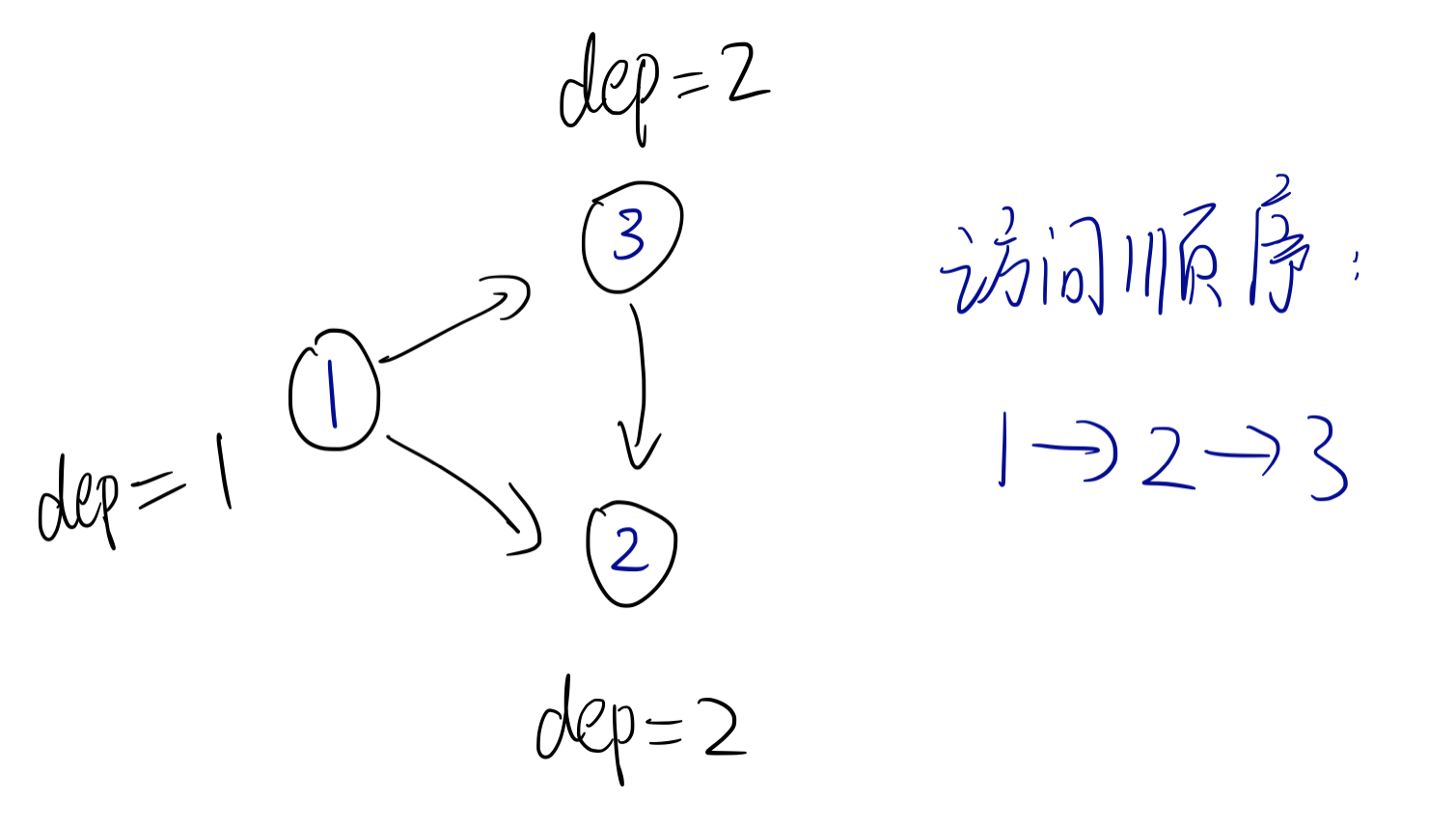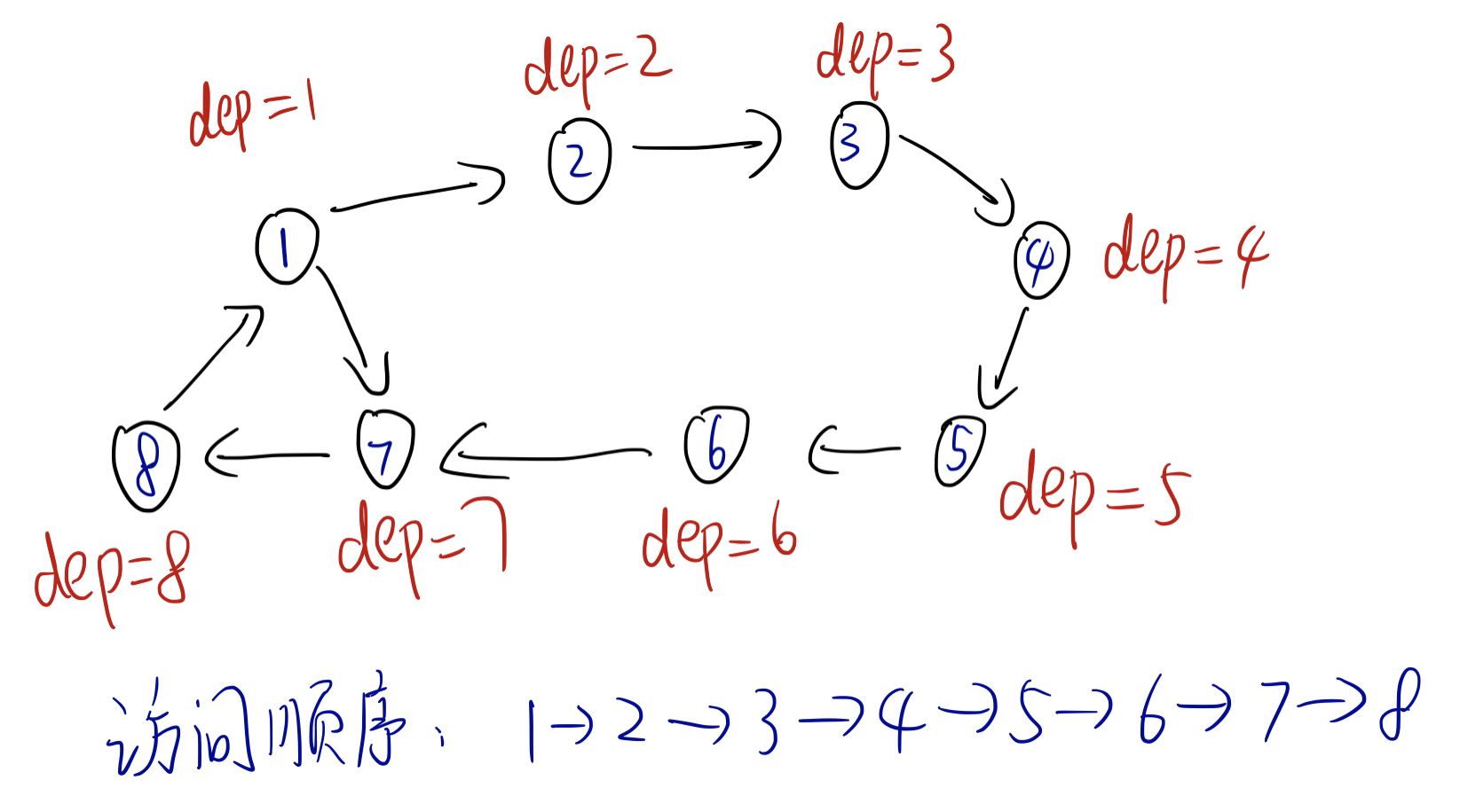## 定义

### 无权无向图求最小环

$n$个vertex, 和一个int $k$, 其中 $3 \leq k \leq n$, 请找出 以下的其中之一:

1. 一个独立集(set of vertex， 两两之间没有edge)， 包含 $\lceil\frac{k}{2}\rceil$ 个vertex

2. 一个simple cycle (set of vertex, 不包含重复vertex)， 其中 $len \leq k$

1. 如果这是一个tree ($m = n-1$), 则 (1)很容易找, 只要dfs一下，做一个图的染色 (染成 $0,1$)即可, 最后取 全部的 $0$ 或者 全部的 $1$

2. 如果不是tree, 必然存在cycle, 那么我们可以找到一个最小环, 最小环必然满足 (1) 或者 (2)！（易证）

•怎么找最小环? 用DFS!

1. 维护一个环的长度 len
2. 维护一个 dep[] 数组, 代表每个vertex的depth
3. 维护一个 pre[] 数组, pre[u] 代表dfs过程中 u的parent
4. 维护一个 int c, 代表找到的cycle的 终点!

1. 从vertex 1开始dfs， dep[to] = dep[cur] + 1 这样来更新 dep[]

2. 当我们找到一个backward edge时, 更新最小环长度

len = min(len, abs(dep[to] - dep[cur]) + 1))


并且更新c, 使得 c = cur, 然后继续探索！

3. dfs结束后, 直接用

vector<int> cycle;
void findcycle() {
while (len--) cycle.push_back(c), c = pre[c];
}


即可找到最小环！

### 无权有向图求最小环

1. $V'$ 是 $V$ 的 non-empty subset
2. $E'$ 是 $E$ 中，所有两端均在 $V'$ 内的edges
3. $V'$ 中，所有的 vertex 的 in-degree 和 out-degree 均为1

1. 维护 ed 代表环的终点，维护最小环长度 final
2. 维护一个 dep[] 数组, 代表每个vertex的depth
3. 维护一个 par[] 数组, par[u] 代表dfs过程中 u的parent
4. 维护一个 in[] 数组，代表在dfs过程中，当前的某个vertex是否存在于递归stack中

dfs过程如下：

int n,m, dep[maxn], par[maxn];
int ans = 1e9, ed = -1, final = 1e8;
vector<int> cycle;
bool in[maxn];

void dfs(int cur) {
in[cur] = 1;
dep[cur] = dep[par[cur]] + 1;
for (int e = head[cur]; e; e = edges[e].nxt) {
int to = edges[e].to;
if (dep[to]) {
if (in[to]) {  // 必须得在递归栈内
int res = abs(dep[cur] - dep[to]) + 1;
if (res < ans) {
ans = res;
ed = cur;
}
}
} else {
par[to] = cur;
dfs(to);
}
}
in[cur] = 0;
}（如上图，如果不考虑 in[] 数组的话，就有可能错误的1->3->2 当作一个环！using namespace std;
#include <bits/stdc++.h>

#define abs(a) ((a>0)?a:-(a))

const int mod = 1e9+7;
const int maxn = 1e3+5;
const int maxm = 2e3+10;

struct Edge {
int to,nxt;
} edges[maxm];

int n,m, dep[maxn], par[maxn];
int ans = 1e9, ed = -1, final = 1e8;
vector<int> cycle;
bool in[maxn];

void add(int u, int v) {
edges[ecnt] = e;
}

void init() {
cin >> n >> m;
for (int i = 1; i <= m; i++) {
int u,v; cin >> u >> v;
}
}

void dfs(int cur) {
in[cur] = 1;
dep[cur] = dep[par[cur]] + 1;
for (int e = head[cur]; e; e = edges[e].nxt) {
int to = edges[e].to;
if (dep[to]) {
if (in[to]) {
int res = abs(dep[cur] - dep[to]) + 1;
if (res < ans) {
ans = res;
ed = cur;
}
}
} else {
par[to] = cur;
dfs(to);
}
}
in[cur] = 0;
}

void renew() {
fill(dep, dep+n+1, 0);
fill(par, par+n+1, 0);
fill(in, in+n+1, 0);
ans = 1e9;
}

int main() {
fastio;
init();

for (int i = 1; i <= n; i++) {
dfs(i);
if (ans < final) {
final = ans;
cycle.clear();
while (ans--) cycle.push_back(ed), ed = par[ed];
}
renew();
}

if (final == 1e8) cout << -1 << endl;
else {
cout << final << "\n";
for (int a : cycle) cout << a << "\n";
}
}


### 有权图求最小环

Floyd $O(n^3)$ 可求！